# Drift and Transport Orderings

It is helpful to define the species-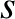toroidal magnetization parameter,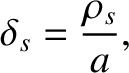(2.110)

the species-poloidal magnetization parameter,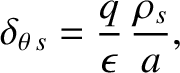(2.111)

and the (modified) species-collisionality parameter,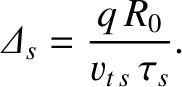(2.112)

As is apparent from Table 2.4, these dimensionless parameters are all much less than unity for both electrons and ions in a tokamak fusion reactor.

In the following, we shall adopt the following fundamental ordering assumptions :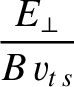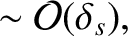(2.113)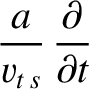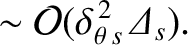(2.114)

Equation (2.113) is known as the drift ordering [18,29], and serves to rule out the rapid plasma motions associated with ideal magnetohydrodynamical instabilities . Equation (2.114) is known as the transport ordering. According to this ordering, any time dependence of plasma quantities is due to the action of perpendicular transport, viscous heating, and frictional heating. The drift and transport orderings, combined with the analysis contained in the following two sections, imply that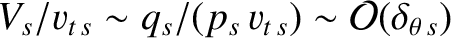,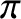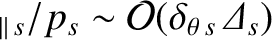,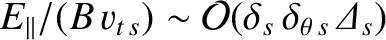,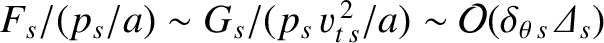, and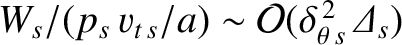.

Equations (2.103)–(2.109) yield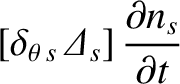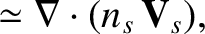(2.115) 0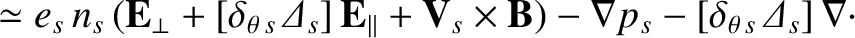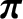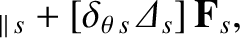(2.116)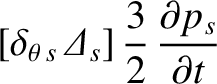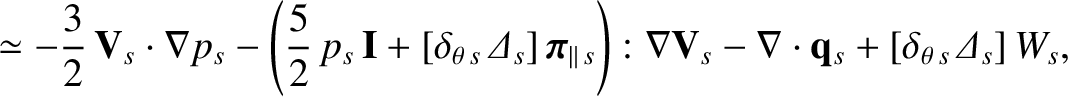(2.117) 0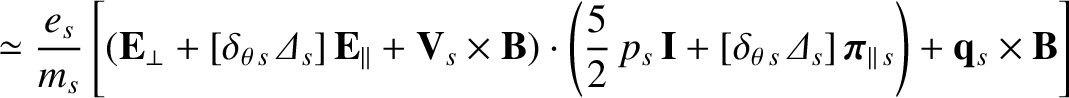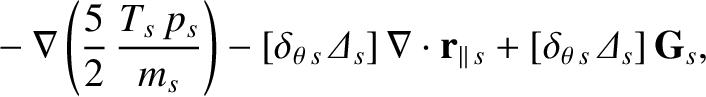(2.118)

where we have written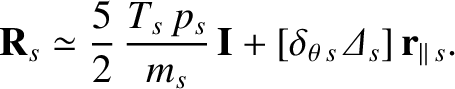(2.119)

In the previous five equations, we have neglected terms that are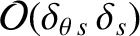or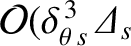) or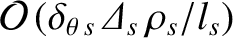smaller than the leading-order terms, while retaining terms that are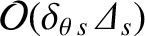, which is only self-consistent if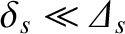. As is shown in Table 2.4, the inequalityis well satisfied for electrons in a tokamak fusion reactor, but is only barely satisfied for ions. The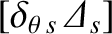factors in the previous equations are there to remind us that the terms they precede aresmaller than the leading-order terms. Finally, it is helpful to define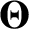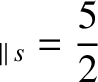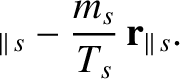(2.120)